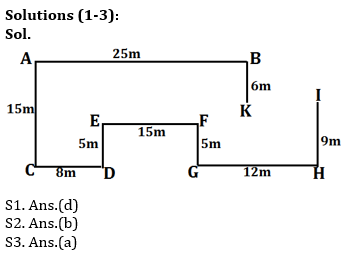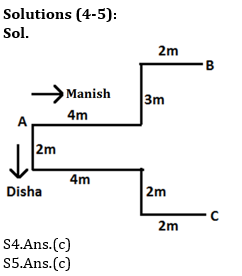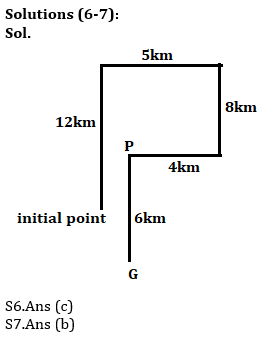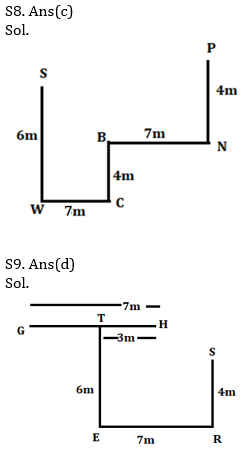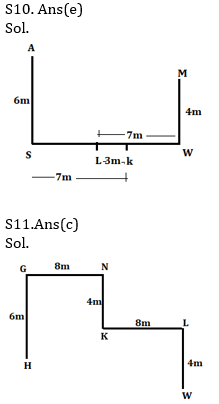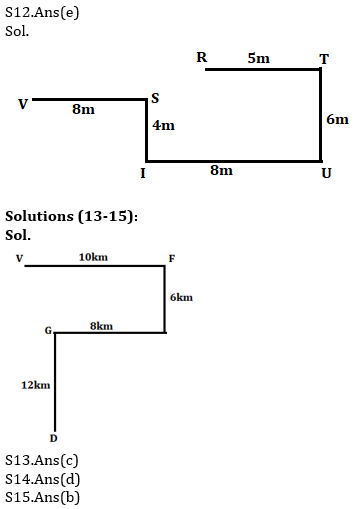Latest Banking jobs   »   Reasoning Ability, Direction Sense Quiz For...

# Reasoning Ability, Direction Sense Quiz For IBPS RRB PO, Clerk Prelims 2021- 16th June

Directions (1-3): Study the following information carefully and answer the given questions:

Point A is 25m west of point B which is 6m north K. Point H is 9m south point I. Point G is 5m south of point F, which is 15m east E. Point C is 15m south of point A. Point H is 12m east of point G. Point E is 5m north of point D, which is 8m east of point C.

Q1. What is direction of point A with respect to point I?
(a) West
(b) North-east
(c) South
(d) North-West
(e) None of these

Q2. What is the distance between point K and point I?
(a) 11m
(b) 10m
(c) 15m
(d) 12m
(e) None of these

Q3. What is the direction of point G with respect to point E?
(a) South-east
(b) West
(c) South
(d) South-west
(e) None of these

Directions (4-5): Read the following information carefully and answer the questions which follow.
Manish, who is facing North, starts from point A, walks 4 m towards the right. He then takes a left turn and walks 3 m before taking a right turn, walking 2 m and stopping at point B. Disha starts from the same point A walks 2 m towards the South. She then takes a left turn and walks 4 m. She now takes a right turn, walks 2 m and then take a left turn and walks 2 m and stopped at point C.

Q4.What is distance between point B and point C ?
(a) 5 m
(b) 8 m
(c) 7 m
(d) 6 m
(e) 9 m

Q5.If Disha walks 4 m towards North from Point C, how far will she be from Point A ?
(a) 4 m
(b) 2 m
(c) 6 m
(d) 8 m
(e) 7 m

Directions (6-7): Study the information carefully and answer the questions given below.
Rakhi started walking 12km in North direction then takes three consecutive right turns and walked distances 5km, 8km, 4km respectively and reached at point P. From point P, she start walking in south direction and walks 6km to reach at point G.

Q6. What is the shortest distance between Initial point and G?
(a) 5km
(b) 4km
(c) √5 km
(d) 2.5km
(e) None of these

Q7. If point R is 2km west of point P then in which direction of point R with respect to initial point?
(a) North
(b) North-west
(c) South
(d) South-west
(e) None of these

Directions (8-10): Each of these questions is based on the following information:
(i) A # M means A is 3m east of M.
(ii) A & M means A is 7m west of M.
(iii) A % M means A is 4m south of M.
(iv) A * M means A is 6m north of M.

Q8. If the expression ‘S*W&C%B&N%P’ is true, what is the direction of W with respect to N?
(a) North-east
(b) South-East
(c) South-west
(d) North-west
(e) None of these

Q9. If the expression ‘G&H#T*E&R%S’ is true, what is the direction of T with respect to S?
(a) West
(b) North-east
(c) East
(d) North west
(e) None of these

Q10. If the expression ‘A*S&K#L&W%M’ is true, what is the shortest distance between M and S?
(a) 13m
(b) 12m
(c) 10m
(d) 14m
(e) None of these

Directions (11-12): Each of these questions is based on the following information:
(i) R & S means R is 5m west of S.
(ii) R % S means R is 8m east of S.
(iii) R * S means R is 6m north of S.
(iv) R @ S means R is 4m south of S.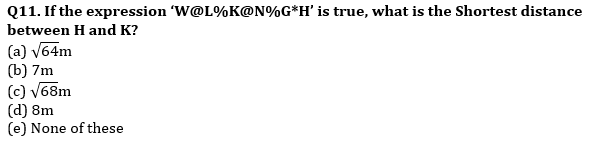Q12. If the expression ‘R&T*U%I@S%V’ is true, what is the direction of U with respect to V?
(a) North west
(b) West
(c) South west
(d) North
(e) None of these

Directions (13-15): Read the following information carefully and answer the given questions:
A man starts walking from point D in north direction after walking 12km he reached at point G then he takes a right turn and covers 8km after that he takes a left turn and covers 6km to reach at point F. Finally, he takes a left turn and walks 10km to reach at point V.

Q13. If point L is 18km south of point V then what is the shortest distance between point D and L?
(a) 5km
(b) 7km
(c) 2km
(d) 4km
(e) None of these

Q14. Find out the shortest distance between point G and point F?
(a) 8km
(b) 9km
(c) 7km
(d) 10km
(e) None of these

Q15. What is the direction of point V with respect to point D?
(a) North-East
(b) North-West
(c) South-East
(d) South-West
(e) None of these

Practice More Questions of Reasoning for Competitive Exams:

###### Study Plan for IBPS RRB PO/Clerk Prelims 2021

Solutions